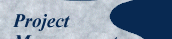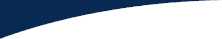## Calculating using Bayes' Theorem

### Case 1: First Major Milestone Met

Because the milestone has been met, the probability of success goes up. Suppose that the pretest probability was 55%. We compute a new number, LR+, which stands for the likelihood ratio for a positive test result. Using the nomogram and the instructions in the Appendix, we find that for e = 0.2 and f = 0.6, the value of LR+ is 2.2.

Then using the same nomogram again, we determine that for a pretest probability of 55% and an LR+ of 2.2, the posttest probability is 73%. Making the first milestone has improved our success probability by 18%, going from 55% to 73%. This is a significant improvement, going from slightly better than even odds to being successful almost three times out of four.

### Case 2: First Major Milestone Missed

Logic dictates that the probability goes down from 55%. Analogously, we compute LR-, which stands for the likelihood ratio for a negative test result. Again using the nomogram and the instructions in the Appendix, we find that for e = 0.2 and f = 0.6, the value of LR- is 0.27. Then using the same nomogram again, we determine that for a pretest probability of 55% and an LR- of 0.27, the posttest probability is about 25%. The probability of success is more than halved, going from 55% to 25%, a drop of 30%. The odds are now three to one against a successful result. Missing the milestone has clearly affected the probability of success.

### Observations

The calculations square with basic logic. The results are asymmetric, in that missing the milestone causes the probability to go down by 30%, whereas making it increases it by only 18%. This asymmetry comes from the 2x2 matrix: False positives are more likely than false negatives, so a positive result buys us less than a negative result costs us.

We started with two parameters, e and f, and computed two new parameters, LR+ and LR-. The information content in both pairs is the same; one pair is a transformation of the other. You will most often encounter LR+ and LR- in the literature. The former ranges from 1 to infinity, with strong tests usually above 10. The latter ranges from 0 to 1, with strong tests closer to zero. Our test is relatively weak: both LR+ and LR- are too close to 1, and the numbers indicate that the test works better in the negative direction.

Finally, where was Bayes' Theorem/Formula/Equation? It is implicit in the nomogram. See the Appendix for more details. Once you had e and f, the subsequent calculations were all performed nomographically. The only required math — arithmetic, really — was computing e and f, each the quotient of two numbers taken from the 2x2 matrix.

### What's Next?

However the test came out, we now have a new baseline for the probability of success, either 25% or 73%. At major milestone #2, we use that number for the pretest probability. It incorporates the original estimate of 55% and the information about the first milestone. You can continue to iterate as often as you choose. Of course, milestone #2 (and all subsequent ones) will have a different 2x2 matrix and a different set of parameters e and f.

Your original estimate need not be super-accurate. The application of a series of tests will usually converge on a value that approaches 100% or 0% as the project nears completion. The rate of convergence depends on the strength of the tests, and the method ensures that we take everything into account. Removing subjectivity from the process is a benefit.